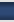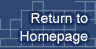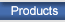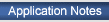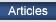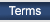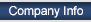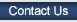The 50 Ohm Story by Dan Doberstein and John Marchettit Nothing is more fundamental to understanding RF and microwave principles than understanding the concept of characteristic impedance. This is one of those topics that gets a lot math thrown at it and very little descriptive talk. Its important to realize from the start that the 50 ohm systems as used in today's RF/microwave systems is a "human made" arbitrary choice. It could have easily been 43 ohms or other numbers, but physical size considerations do dictate the range of practical coax cables to be in the 20 to 200 ohm range. Just as important is to remember that the characteristic impedance concept is so broad as to include all types of coaxial lines, printed circuit traces (microstrip/stripline) , twin lead and twisted pair. Remarkably free space itself has an impedance. In the case of free space and other unbounded medium this impedance is called the intrinsic impedance. So, someone hands you a 1000 foot roll of coax cable and says to you " that's 50 ohm coax use it wisely". You decide check out this "50 Ohm" assertion. With your ohmmeter on one end from center conductor to outer conductor (no connection at other end)  you are surprised  to see it reads near infinite impedance! You then short one end and measure the open end with the meter. It now reads near zero ohms! " How can this be!" you ask yourself , "I was assured it was 50 ohm cable!" The reason your meter did not tell you that the cable was 50 ohms is that it could NOT read the Instantaneous voltage/current ratio( V=IR) and its own internal resistance is so high that it causes a very large time constant in the reading. You cannot use a typical ohmmeter to measure characteristic impedance. So how can we measure the characteristic impedance of your coax? Instead of trying to use an ohmmeter we will use the circuit of figure 1. The circuit allows us to generate a pulse of current by toggling the switch with the asterisk indicating where one would measure the current. Now what happens? At the moment the switch connects battery (+) to the center conductor of the coax cable it starts to "charge up" that piece of coax, sort of like charging a capacitor. Then we discharge the cable by shorting center conductor to shield or battery minus. This provides our "pulse" of current. If you measure the current in the center conductor during the pulse generation it will reach a maximum value of Imax=Vbat / Zo, where Zo is the characteristic impedance or equivalently called the surge impedance of the coax. The question arises what properties of coax limit the inrush current to the expression given above? Or stated another way why doesn't the coax charge up "instantly"? To answer that question let's examine the way an ideal capacitor would charge up vs. coax. In theory , an ideal , discharged, capacitor would see an infinite current for zero time if you connect it to a perfect source ( a perfect source has zero internal resistance). Stated another way it charges up "instantly" to the applied voltage of the source. There are two crucial differences in the way a piece of coax charges up and a ideal capacitor would charge up when connected to a battery. First an ideal capacitor has zero inductance in the current path, which would limit current inrush rate. Second an ideal capacitor has zero physical length so no there is no propagation in space of the current pulse. Coax cable does not charge up instantly. This is because it has a finite series inductance per unit length, a capacitance per unit length and it has a physical length which all contribute to a propagation distribution in time and space of the current surge. In short the series inductance resists the flow of current that wants to charge the capacitance therefore causing propagation delay of the current surge. This propagation delay causes current surge to spread in time. Simultaneously the physical length creates a propagation distribution in space of the current surge. So instead of a infinite "impulse" of current in zero time and zero space, as in the ideal capacitor, the current quickly rises to a maximum and starts propagating down the coax. The speed of propagation is always less than the speed of light and depends on the materials the coax is made from. The current surge method is not how one would normally measure coax cable characteristic impedance but it is a viable method and has an intuitive appeal. Another way to measure the characteristic impedance of coax cable is to measure its inductance and capacitance per unit length , the square root of L divided by C will be in ohms (not farads or henrys) and will be equal to the characteristic impedance. Why do different cables have different characteristic impedances? Every coax cable or other transmission media has its own unique capacitance and inductance per unit length. For coax cables this will be determined by inner/outer conductor ratios and the dielectric constant of the material between conductors for coax cables. For microstrip lines its primarily the trace width, dielectric constant of the pc board and thickness of PC board. Okay, so now maybe "50 Ohm" cable makes some sense and you are now a "50 ohm" systems zealot. You now strive for "perfect 50 ohms" in all your 50 ohm system work. You have become so unreasonable that you insist that all systems be EXACTLY 50 ohms. Now you are in trouble. In truth no coax, connector, amplifiers etc. is EXACTLY 50 ohms. The fact is that it is amazing how far off 50 Ohms you can be in your designs and not see that much degradation in performance! We need a way of expressing how close we are to 50 Ohms in our designs and systems. The most common way of doing this is VSWR or Voltage Standing Wave Ratio. So before we can discuss your fervent pursuit of "pure" 50 ohm systems we need to understand the concept of VSWR. In fact  VSWR calculations will work for ANY characteristic impedance, 50 ohms or otherwise. Now suppose you took your 1000 foot roll of 50 ohm coax and cut a 20 foot chunk off it. Now connect one end to a real nice RF type signal generator that we will assume has perfect "50 ohm" impedance and leave the other end of the coax chunk open. Set the generator frequency to say 50 MHz , although just about any frequency will work, 50 MHz is a good spot for most coax. So now we have our "50 ohm " generator supplying a 50 MHz sinewave to a "50 Ohm" piece of coax with no connection at the other end. What happens? Well here is what happens: The sinewave when FIRST applied to the cable, starts to "propagate" towards the open end of the cable, just like our pulse did. When the sinewave gets to the end of the cable it COMPLETELY "reflects" , turns around, and heads right back towards the generator! Once inside the generator it "dissipates" itself as heat in the generators internal 50 ohm resistor. Now we do the same experiment except we short the other end of the coax cable. Again we would see a complete reflection of the sinewave and total dissipation of reflected wave within the internal 50 ohms of the generator ( there will be a phase reversal wrt to open case). So, if the cable end is open or shorted we get TOTAL reflection of our applied sinewave. This is defined as a VSWR of "infinity to1" Now we connect a "perfect" 50 ohm resistor at the end of the coax line. In this case we TERMINATED the cable in its characteristic impedance. The applied sinewave will COMPLETELY dissipate in this termination and there will be zero reflection back towards the generator. Why? Because we fooled the sinewave into thinking it was traveling down a "infinite" piece of cable therefore no reflection. This condition has the LOWEST VSWR obtainable and is defined as 1 to 1 or written usually as 1:1. A VSWR of 1:1 for a coax cable termination means it is exactly equal to the characteristic impedance and we will have ZERO reflection from this termination. A closely related parameter is the reflection coefficient. This term not only records the magnitude of the reflected wave but also its angle. Because the reflection coefficient measures magnitude of reflection and its angle it is VECTOR measurement. VSWR only measures magnitude and is therefore a SCALAR measurement. The VSWR can be calculated if the Reflection coefficient is known, see below. The table also shows Return loss and Mismatch loss. Return loss (RL) is a measure of how much power is reflected from a load or termination. The closer the termination or load is to "ideal" characteristic impedance the lower the reflected power. It is expressed in dB referenced to the incident power and is usually negative indicating a lower power reflected than absorbed by load. Again VSWR can be calculated if RL is known. Any RL that is better than -15dB is usually considered quite acceptable. Mismatch Loss (ML) indicates how much power is lost when signal travels from one characteristic impedance to another. Since no system of connectors is perfect ML occurs at every connector, connection etc. Okay back to the real world. There are no perfect terminations and there are no perfect 50 ohm resistors. Let's examine what happens when we use a real world termination on coaxial cables, one that's slightly off or in some way imperfect. Suppose you were working on a 50 ohm system and you needed to terminate a open coax cable end to prevent unwanted reflections. Unfortunately the only terminations you have in your pocket are all 75 ohm type. Assuming you can get the connector to mate what will happen if you terminate that 50 ohm line with a 75 ohm termination? First off 75 ohms is pretty darn close to 50 ohms. If you use the formulas in the table below you will calculate a VSWR of 1.5:1 . But because it is not exactly 50 ohms some of the sinewave or signal WILL be reflected back towards generator. But not very much. A VSWR of 1.5:1 is quite respectable and if you calculate the reflected power you will see it is small, almost 14 dB down from applied! Many commercially available discrete RF amplifiers (MMIC'S) barely achieve or have worse than 1.5:1 VSWR's and these are claimed to be "50 ohm" system parts! We now hope that your devotion to 50 ohms is getting more tolerance in it. Below is another real world example of how one can cheat a bit on characteristic impendence "rules" and get away with it. Example Problem, a Satellite TV IF Cable run: A satellite TV system typically uses 75 ohm coax after the LNA/Block downconverter(LNB) . In this installation a 50 foot run of coax is need between the LNB and IF Decoder unit. It is desired to use a small light weight 50 ohm coax instead of heavier , larger diameter 75 coax. What effect will this have on system performance or stated more precisely, What is the system effect of a coaxial miss-match of 50 ohms to 75 ohms?Based on the above figures, this data supports that a change in impedance to 50 ohm coax in the coax feed to the IF decoder that has a source impeadance of 75 ohms is minimal. Its also important to know that in this case the IF decoder receives a signal that has been translated to a much lower frequency with a lot of gain "upfront" in the LNB block. That gain does two things , sets the noise figure of the system at the LNB and provides isloation to downstream reflections. The net effect is that even if some power is lost due to mismatch loss we have plenty to spare from the high gain upfront. As for the reflected signal the high isolation of the LNB protects the system from being adversly affected.Back to top